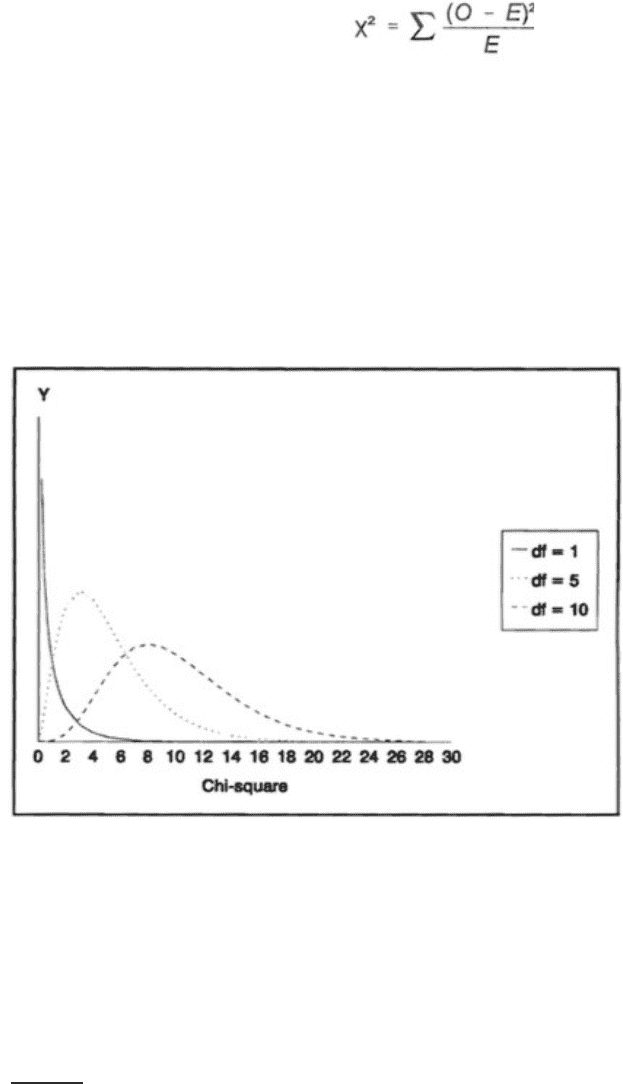Textbook Notes (290,000)
CA (170,000)
U of G (10,000)
PSYC (3,000)
Chapter 8

# PSYC 2040 Chapter Notes - Chapter 8: Chi-Squared Test, Chi-Squared Distribution

Department
Psychology
Course Code
PSYC 2040
Professor
Naseem Al- Aidroos
Chapter
8

This preview shows page 1. to view the full 4 pages of the document.ch 8- chi square (summary)
chi square testing is used when determining differences in frequency of events
tries to find an association/ contingency/ correlation between factors of interest
compares the expected frequencies with obtained frequencies and determines the
goodness of fit of the data to the model
-ex: the frequency of smoking vs non- smoking and the relation between gender
X2 (chi-square) statistic:
O = the obtained frequency
E = the expected frequency
the sampling distribution is approximated by the density function for X2
with degrees of freedom equal to the number of obtained frequencies that are free to
vary given whatever restrictions imposed provided that the null hypothesis is true.
null hypothesis: that in the population the observed frequencies equal the expected frequencies.
expect a given amount of variability based on sampling
if this variability is greater than what would be expected on the basis of chance, then
one would conclude that the null hypothesis is not true
this is evaluated using the T distribution or the F-distribution.
With 1 degree of freedom, the
distribution is exponential
df 5- shows as an
asymmetrical distribution with
a long tail running to the right
df-10, the distribution is
somewhat less skewed, but
nonetheless asymmetrical.
the mean of the chi-square
distribution increases (the
centre of the distribution
moves to the right) as the
degrees of freedom increase.
as df increases, the value required for significance also increases.
For 1 df, a value of 3.84 is required for significance at the .05 level, and a value of 6.84 is
required at the .01 level.
the X2 statistic is not a parametric distribution
it’s the ratio of the sum of squared deviations of n observations from their sample mean
divided by the population variance.
History